Shallow Foundations - 2

# Shallow Foundations - 2 - Notes | Study Foundation Engineering - Civil Engineering (CE)

 1 Crore+ students have signed up on EduRev. Have you?

Effect of Water Table on Bearing Capacity of Soil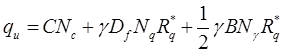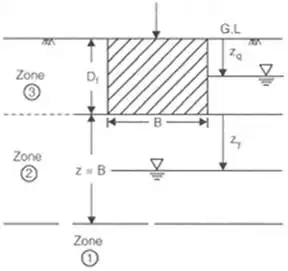where Rq* and Ry* are water table correction factor.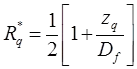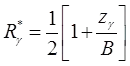when 0 ≤ zq ≤ Df when 0 ≤ zγ ≤ B.
If Zγ > B they Rγ= 1
If Zγ ≤ 0 they
If water table rise to G.L
Rq* = 1 / 2 and Rγ* = 1 / 2

1. Significant only for cohesionless.
2. Short duration test hence only results in immediate settlement.
(i) quf / qup = Bf / Bp
(ii) quf = qup
..for ∅ = soil … for C-soil
If plate load test carried at foundation level then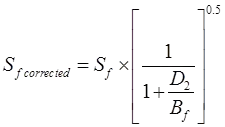(iii)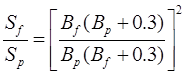(iv) Sf / Sp = Bf / Bp
… for dense sand.  … for clays
(v) Sf / Sp = (Bf / Bp)n + 1
… for silts.
where,
quf =Ultimate bearing capacity of foundation
qup = Ultimate bearing capacity of plate
Sf = Settlement of foundations
Sp = Settlement of plate
Bf = Width of foundation in m
Bp = Width of plate in m
Housels Approach
QP = mAP + nPP
Qf = mA+ nPf
where, Qp = Allowable load on plate m and n are constant
P = Perimeter Ap = Area of plate
Af = Area of foundation

Standard Penetration Test

Significant for Granular Soils

1.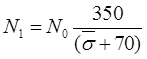and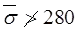where, N1 = Overburden pressure correction
N0 = Observed value of S.P.T. number.
= Effective overburden pressure at the level of test in kM/m2.
2. For Saturated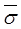fine sand and silt, when N1 > 15
N2 = 1/2(N1 - 15) + 15
where, N2 = Dilatancy correction or water table correction.
Nq + Nγ related to N value using peck Henson curve or (code method)
Teng's formula relate N value with reading capacity of granular soil.

Pecks Equation
qa net = 0.44NS = CwkN/m2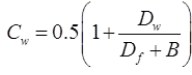Dw = depth of water table below G.L
Df = Depth of foundation
B = Width of foundation
N = Avg. corrected S.P.T. no.
S = Permissible settlement of foundation
Cw = Water table correction factor
qa net = Net allowable bearing pressure.

Teng's Equations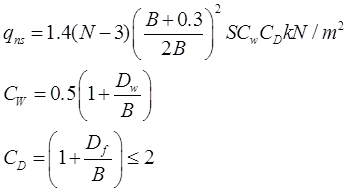Cw =Water table correction factor
Dw = Depth of water table below foundation level
B = Width of foundation
Cd =Depth correction factor
S = Permissible settlement in 'mm'.

I.S Code Method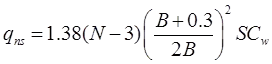qns =Net safe bearing pressure in kN/m2
B = Width in meter.
S = Settlement in 'mm'.

I.S. Code Formula for Raft

qns = 0.88NSCw

Cw: Same as of peck Henson.

Meyer-Hoffs Equation

qns = 0.88NSCwCd
where, qns = Net safe bearing capacity in kN/m2.
B < 1.2 m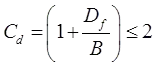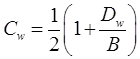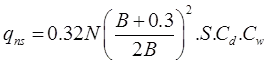B ≥ 1.2 m (where qns is in kN/m2.

Cone Penetrations Test

1.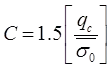where, = Static cone resistance in kg/cm2c = Compressibility coefficient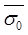= Initial effective over burden pressure in kg/cm2.
2.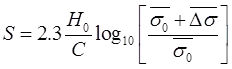where, 'S' = Settlement.
3. qns = 3.6qsRw B > 1.2 m.where, qns = Net safe bearing pressure in kN/m2.
4. qns = 2.7qc.Rw B < 1.2 m.where, Rw = Water table correction factor.
The document Shallow Foundations - 2 - Notes | Study Foundation Engineering - Civil Engineering (CE) is a part of the Civil Engineering (CE) Course Foundation Engineering.
All you need of Civil Engineering (CE) at this link: Civil Engineering (CE)

## Foundation Engineering

18 videos|27 docs|20 tests
 Use Code STAYHOME200 and get INR 200 additional OFF

## Foundation Engineering

18 videos|27 docs|20 tests

Track your progress, build streaks, highlight & save important lessons and more!

,

,

,

,

,

,

,

,

,

,

,

,

,

,

,

,

,

,

,

,

,

;